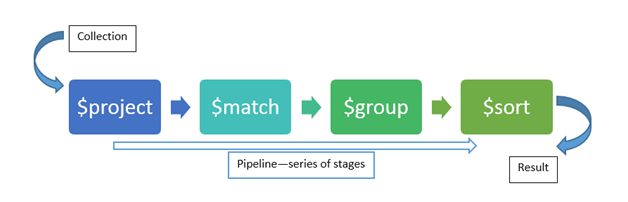Full stack developer

## Find the median from data series using MongoDB aggregation queryMongoDB’s aggregation is very powerful. It works as a pipeline where your data passes through each “stage” and each of those stages modifies the data. You can filter, group, sort, and much more in those stages. MongoDB’s aggregation supports a lot of inbuild operators for common operations. Like `\$avg`, `\$sum` for doing basic mathematical operations. However, there isn’t one for calculating a “median”.

A few days back, I was working on generating some data for an API that was supposed to be used by a graphing library. The API was required to give a median of a given property from the collection between a given date range. To calculate the median, I had to build a multi-stage pipeline. I ended up with these stages,

1. Match - `\$match`
2. Group - `\$group`
3. Sort - `\$sort`
4. Project - `\$project` (as you will see below, we will have to project our data multiple times before getting the desired result.)

First, we need to filter the data based on given date range. So the query would be,

``````db.collection.aggregate([
{ "\$match": { "startDate": { "\$gte": "\$startDate" }, "endDate": { "\$lte": "\$endDate" } } }
])
``````

Now, we need to sort all records them in ascending order by value. Remember, the median is calculated by sorting the values and then picking up the value that is at the midpoint.

``````{ "\$sort": { "value": 1 } } // 1 is ascending and -1 would be descending
``````

Next, we will group the data and collect all the values of the required property into an array (you will later understand why we need this)

``````{
"\$group": {
"_id": "\$userId" // assuming the values are required to be group by user
"valueArray": {
"\$push": "\$value" // this is the property for which the median needs to be calculated
}
}
}
``````

To find the midpoint i.e. the element in the center of the array, we need to find its index. To find the index, we need to first find the length of the array. For that, we will use `\$project` operation and project our data to include an array size property

``````{
"\$project": {
"_id": 0, // the _id is the group key from the previous group operation and by specifying '0' here we say that we don't want this value anymore
"userId": "\$_id", // we removed the _id and renamed it to userId
"valueArray": 1,
"size": { "\$size": [ "\$valueArray" ] }
}
}
``````

Now that we have the size of the array, let’s find the midpoint index. But, finding the midpoint index is easy if the length of the array is odd. For example, for an array `[1, 2, 3]` the mid point is `2`. But what if the array length is an even number? For example, to get the median for this array `[1, 2, 3, 4]` we need to find two indexes in the middle, get the value, sum them up, and then divided them by two. So for the array, the median would be

`2 + 3 = 5 / 2 = 2.5`

The next thing we need to do is to find out whether our array is an odd or even length array. For that, we will do another `\$project` operation.

``````{
"\$project": {
"userId": 1,
"valueArray": 1,
"isEvenLength": { "\$eq": [{ "\$mod": ["\$size", 2] }, 0 ] },
"middlePoint": { "\$trunc": { "\$divide": ["\$size", 2] } }
}
}
``````

If the array is even length we need to find the middle point range (the value `2` and `3` in the above example)

``````{
"\$project": {
"userId": 1,
"valueArray": 1,
"isEvenLength": 1,
"middlePoint": 1,
"beginMiddle": { "\$subtract": [ "\$middlePoint", 1] },
"endMiddle": "\$middlePoint"
}
}
``````

Now that we have the indexes, let’s get the actual value from the array

``````{
"\$project": {
"userId": 1,
"valueArray": 1,
"middlePoint": 1,
"beginMiddle": 1,
"beginValue": { "\$arrayElemAt": ["\$stepsArray", "\$beginMiddle"] },
"endValue": { "\$arrayElemAt": ["\$stepsArray", "\$endMiddle"] },
"isEvenLength": 1
}
}
``````

Once we have the sum, for the even length array, let’s calculate the average for values in the middle point range

``````{
"\$project": {
"userId": 1,
"valueArray": 1,
"middlePoint": 1,
"beginMiddle": 1,
"beginValue": 1,
"endValue": 1,
"middleSum": { "\$add": ["\$beginValue", "\$endValue"] },
"isEvenLength": 1
}
}
``````

and finally, based on the length of the array, we get the median value

``````
"\$project": {
"userId": 1,
"valueArray": 1,
"median": {
"\$cond": {
if: "\$isEvenLength",
then: { "\$divide": ["\$middleSum", 2] },
else:  { "\$arrayElemAt": ["\$stepsArray", "\$middlePoint"] }
}
}
}
}
``````

The whole pipeline with stages looks like this,

``````db.collection.aggregate([
{ "\$match": { "startDate": { "\$gte": "\$startDate" }, "endDate": { "\$lte": "\$endDate" } } },
{
"\$group": {
"_id": "\$userId",
"valueArray": {
"\$push": "\$value"
}
}
},
{ "\$sort": { "value": 1 } },
{
"\$project": {
"_id": 0,
"userId": "\$_id",
"valueArray": 1,
"size": { "\$size": ["\$valueArray"] }
}
},
{
"\$project": {
"userId": 1,
"valueArray": 1,
"isEvenLength": { "\$eq": [{ "\$mod": ["\$size", 2] }, 0 ] },
"middlePoint": { "\$trunc": { "\$divide": ["\$size", 2] } }
}
},
{
"\$project": {
"userId": 1,
"valueArray": 1,
"isEvenLength": 1,
"middlePoint": 1,
"beginMiddle": { "\$subtract": [ "\$middlePoint", 1] },
"endMiddle": "\$middlePoint"
}
},
{
"\$project": {
"userId": 1,
"valueArray": 1,
"middlePoint": 1,
"beginMiddle": 1,
"beginValue": { "\$arrayElemAt": ["\$stepsArray", "\$beginMiddle"] },
"endValue": { "\$arrayElemAt": ["\$stepsArray", "\$endMiddle"] },
"isEvenLength": 1
}
},
{
"\$project": {
"userId": 1,
"valueArray": 1,
"middlePoint": 1,
"beginMiddle": 1,
"beginValue": 1,
"endValue": 1,
"middleSum": { "\$add": ["\$beginValue", "\$endValue"] },
"isEvenLength": 1
}
},
{
"\$project": {
"userId": 1,
"valueArray": 1,
"median": {
"\$cond": {
if: "\$isEvenLength",
then: { "\$divide": ["\$middleSum", 2] },
else:  { "\$arrayElemAt": ["\$stepsArray", "\$middlePoint"] }
}
}
}
}
])
``````

I hope this helps.

Happy coding!

PS: I have also answered a question on Stack overflow with this same aggregation query.